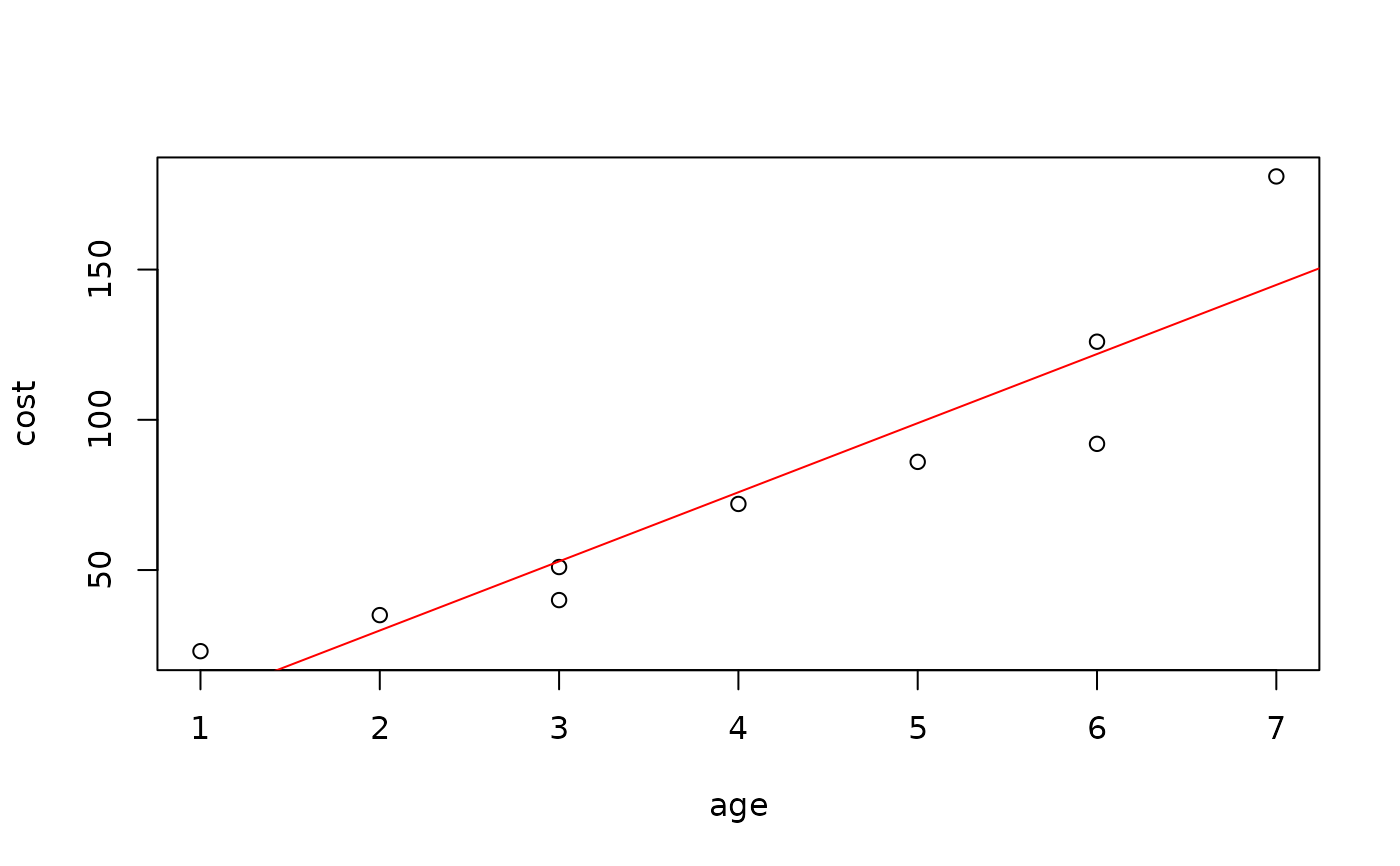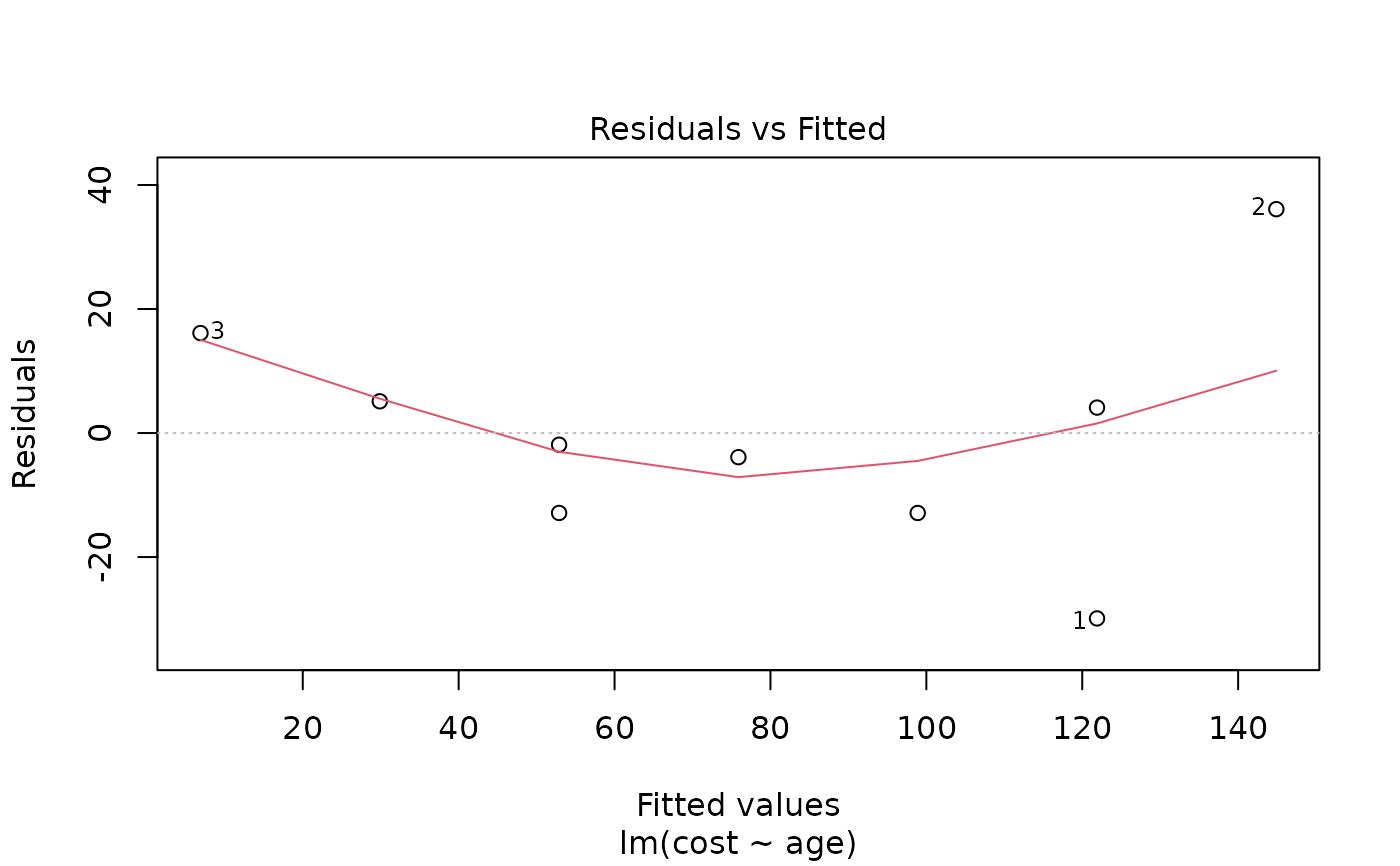Data for Exercise 2.3, 2.39, and 2.54

Register

## Format

A data frame/tibble with nine observations on two variables

age

age of cash register (in years)

cost

maintenance cost of cash register (in dollars)

## Examples


plot(cost ~ age, data = Register)
model <- lm(cost ~ age, data = Register)
abline(model, col = "red")predict(model, newdata = data.frame(age = c(5, 10)))
#>         1         2
#>  98.89189 213.90878
plot(model, which = 1)rm(model)